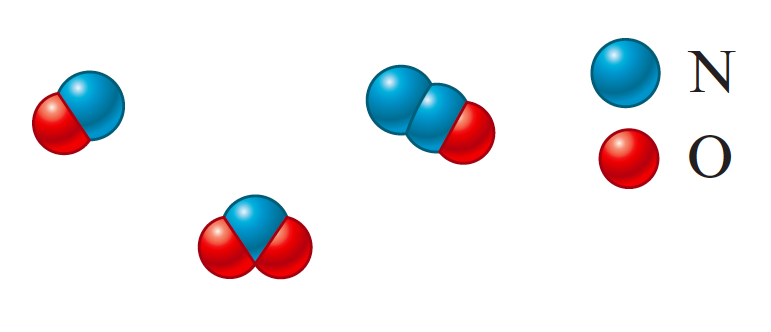# Problem: The percent by mass of nitrogen for a compound is found to be 46.7%. Which of the following could be this species?

🤓 Based on our data, we think this question is relevant for Professor Fakhreddine's class at TEXAS.

###### FREE Expert Solution

Based on the given, the compound is composed of nitrogen and oxygen only

% Nitrogen = 46.7%

% Oxygen = 100% - 46.7% = 53.3%

Assume 100 g of sample:###### Problem Details

The percent by mass of nitrogen for a compound is found to be 46.7%. Which of the following could be this species?